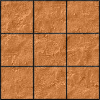#### You may also like### Pyramids

What are the missing numbers in the pyramids?### Paving the Way

A man paved a square courtyard and then decided that it was too small. He took up the tiles, bought 100 more and used them to pave another square courtyard. How many tiles did he use altogether?### Chess

What would be the smallest number of moves needed to move a Knight from a chess set from one corner to the opposite corner of a 99 by 99 square board?

# Reversals

##### Age 11 to 14 Challenge Level:

This problem is available as a printable worksheet: Reversals.pdf

### Why do this problem?

This problem strengthens students' understanding of place value and can help them to appreciate the power of algebra for solving number problems.

### Possible approach

You may wish to work on the problems Always a Multiple and Special Numbers first.

Introduce the first problem:
"I chose a two-digit number, divided it by 2, multiplied the answer by 9, and then reversed the digits. My answer was the same as my original number. Can you work out what my number was?"
Give students time to think about the problem and discuss it with their neighbour. Then share approaches. If no-one has used algebra, introduce the idea of representing a two-digit number as 10a + b.

Next, give students the other three problems:

• I chose a two-digit number, added 1, divided the answer by 2, and then reversed the digits. My answer was the same as my original number! Can you find the number I chose?
• I chose a two-digit number, subtracted 2, divided the answer by 2, and then reversed the digits. My answer was the same as my original number! What was my number?
• Choose a number, subtract 10, divide by 2 and reverse the digits. What number should you start with to get an answer the same as your original number?
Bring the class together and check everyone has successfully used an algebraic method to find the solutions. If appropriate, invite students to try some of the extension problems.

### Possible extension

Choose a 3-digit number where the last two digits sum to the first (e.g. 615).
Rotate the digits one place, so the first digit becomes the last (so for the example, we get 156).
Subtract the smallest number from the largest and divide by 9 (which is always possible).
What do you notice about the result? Can you explain why?

These problems can all be solved using similar techniques:

### Possible support

Always a Multiple provides a geometric as well as an algebraic way of thinking about place value related number puzzles, and might be a useful introduction to the ideas used in this problem.